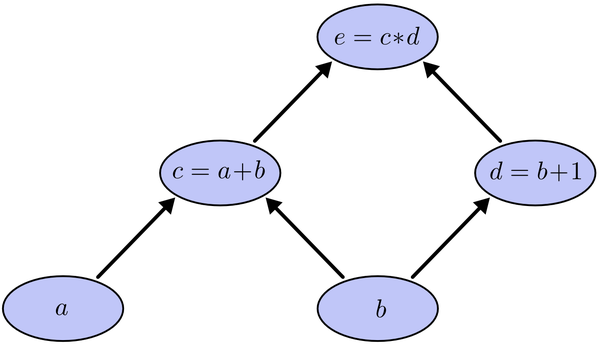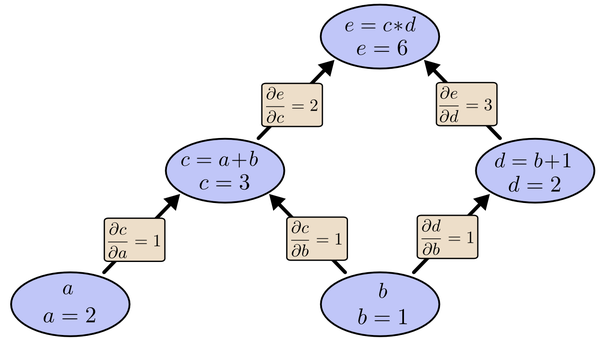# 深度学习：深度前馈网络

## 二 示例：学习XOR

### 2.1 模型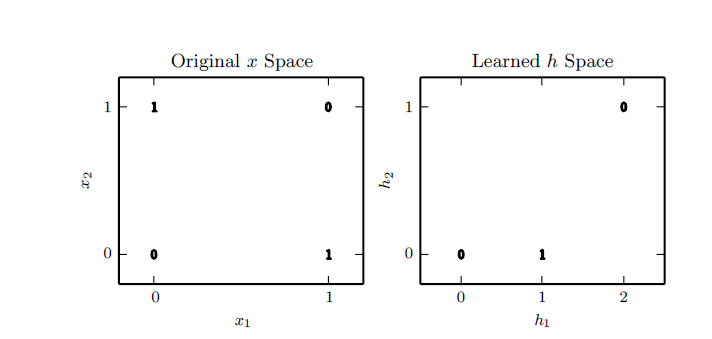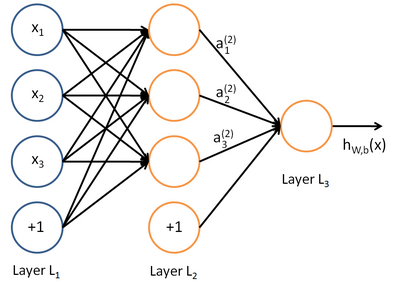### 2.2 ReLU激活函数

ReLU作为激活函数的依据是，生物的稀疏激活性。人脑神经元同时被激活的只有1%-4%，这可提高学习精度，更好更快地提取稀疏特征。ReLU的非线性来源于神经元的部分选择性激活。

## 三 基于梯度的学习

### 3.1 损失函数

• 非负性
• 期望与实际误差小时，损失值小，反之则大。

### 3.2 输出神经元

#### 3.2.3 多重Bernoulli分布Softmax输出神经元

softmax是sigmoid函数的普遍形式，用于多分类。用在输出层，作为一个分类器，表述为多个分类上的概率分布。 softmax函数的常见形式如下：

## 3.3 隐藏神经元

### 3.3.1 Relu神经元和其泛化

Relu激活函数，全面

Relu，更学术，前沿

Relu线性神经元的激活函数为 $g(z)=max\lbrace 0,z \rbrace$ 。

Relu与线性神经元很像，唯一的不同是，Relu的输出取值范围有一半为0（非激活）。这使得其导数一直很大，梯度不仅大而且连续。激活的神经元的二阶导数几乎处处为0，一阶导数处处为1。

Relu一般用在外层映射，比如 $h=g(W^Tx+b)$ 。初始化Relu时，建议取较小的b，这会使得Relu对大部分输入都是激活的。

• 绝对值Relu:令 $\alpha_i=-1$，那么 $g(z)=\mid z\mid$ 。可以用在图像识别中寻找极性反转时特征不变的特征。

• leaky Relu:令 $\alpha =0.001$ 取一个较小值。

• 参数化Relu:将 $\alpha$ 作为一个可以学习的参数。

## 3.4 其他隐藏神经元

• Radial basis function（RBF）：$h_i =exp(-\frac{1}{\sigma i^2\mid \mid W-x\mid \mid ^2})$ 。只有当x接近于模板 $W_{:,i}$ 时函数才会被激活，由于其对大多数的x都是饱和状态，所以很难优化。

• softplus ：$g(a)=\varsigma (a)=log(1+e^a)$ 是Relu的平滑版本，通常不鼓励使用softplus。

• Hard tanh：形状与tanh和Relu很像，但是与Relu不同的是，它是有界的。 $g(a)=max(-1,min(1,a))$ 。

## 3.5 网络架构设计

### 3.5.1 通用近似属性和深度

• 用来训练的优化函数，可能无法找到拟合目标函数的正确参数。
• 训练算法有可能因为过拟合选择错误的函数。

### 3.5.2 其他架构考虑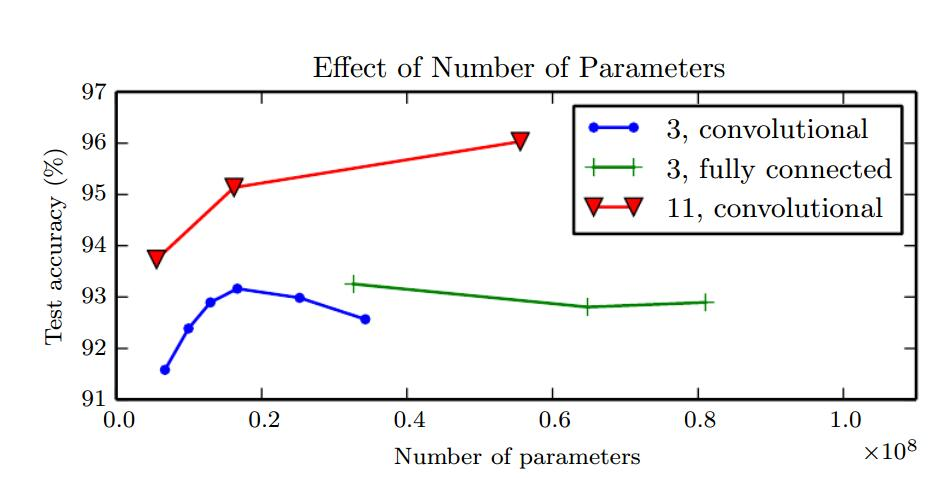## 4 反向传播算法